# Bombieri prime number theorem

(diff) ← Older revision | Latest revision (diff) | Newer revision → (diff)

For many important problems in the theory of numbers one needs information about the average distribution of prime numbers in arithmetic progressions (cf. also Number theory; Prime number; Dirichlet theorem). Let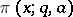denote the number of primes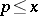satisfying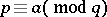. One looks for inequalities of the type (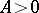arbitrary but fixed)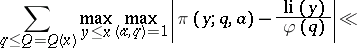(a1)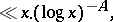where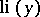is the logarithmic integral (cf. also Distribution of prime numbers) andis the Euler totient function (cf. Totient function).

The first attempt to obtain a "non-trivial" estimate of this kind was made by A. Rényi in 1948. He showed that (a1) is true with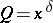for some small positive. Due to subsequent refinements of M.B. Barban, Pan Cheng Dong, A.I. Vinogradov and, finally, E. Bombieri it is known that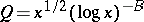for some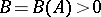. Somewhat later, P.X. Gallagher introduced major simplifications in Bombieri's arguments. More recently, R.C. Vaughan developed an ingenious new method which gives a still simpler proof by essentially elementary means; a general reference is [a2].

The critical exponent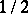in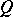can conjecturally be replaced by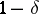(the Halberstam conjecture). Under certain restrictive conditions, Fouvry–Iwaniec and Bombieri–Friedlander–Iwaniec have given refinements toand; see [a1].

The main advantage of Bombieri's theorem becomes clear by noting that the classical Page–Siegel–Walfisz prime number theorem (cf. Page theorem) only leads to the limit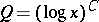for the moduli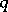in (a1). Moreover, Bombieri's boundis as good, apart from the logarithmic factor, as one can obtain on the assumption of the generalized Riemann hypothesis (cf. Riemann hypotheses). This makes it often possible to circumvent the use of the extended Riemann hypothesis, which has far-reaching implications in number theory; for example, it gives approaches to such important results as the Titchmarsh divisor problem, the Hardy–Littlewood formula for the number of representations of an integer as a sum of a prime and two squares or Chen's celebrated theorem that every sufficiently large even integer is the sum of a prime and an almost-prime having at most two prime factors; a general reference is [a3].

Bombieri's result has also been generalized to algebraic number fields, by a number of scientists. There are various ways in which this can be done, but the principle underlying the treatment is always that of the large sieve. In view of applications Hinz's multi-dimensional version of Bombieri's theorem is of some interest, see [a4]. This leads, among other things, to an analogue of Chen's theorem in totally real fields, see [a5].